Courses

# NCERT Solutions(Part - 3) - Perimeter and Area Class 7 Notes | EduRev

## Mathematics (Maths) Class 7

Created by: Praveen Kumar

## Class 7 : NCERT Solutions(Part - 3) - Perimeter and Area Class 7 Notes | EduRev

The document NCERT Solutions(Part - 3) - Perimeter and Area Class 7 Notes | EduRev is a part of the Class 7 Course Mathematics (Maths) Class 7.
All you need of Class 7 at this link: Class 7

EXERCISE 11.4

Question 1: A garden is 90 m long and 75 m broad. A path 5 m wide is to be built outside and around it. Find the area of the path. Also find the area of the garden in hectares.

Length of rectangular garden = 90 m and breadth of rectangular garden = 75 m

Outer length of rectangular garden with path = 90 + 5 + 5 = 100 m

Outer breadth of rectangular garden with path = 75 + 5 + 5 = 85 m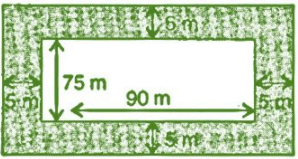Outer area of rectangular garden with path = length x breadth = 100 x 85 = 8,500 m2

Inner area of garden without path = length x breadth = 90 x 75 = 6,750 m2

Now, Area of path = Area of garden with path – Area of garden without path

= 8,500 – 6,750

= 1,750 m2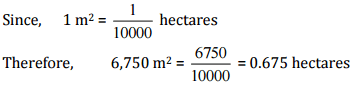Question 2:

A 3 m wide path runs outside and around a rectangular park of length 125 m and breadth 65 m. Find the area of the path.

Length of rectangular park = 125 m

Breadth of rectangular park = 65 m

Width of the path = 3 m

Length of rectangular park with path = 125 + 3 + 3 = 131 m

Breadth of rectangular park with path = 65 + 3 + 3 = 71 m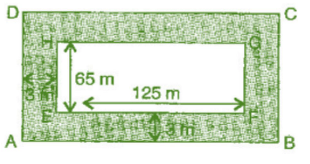∴ Area of path = Area of park with path - Area of park without path
= (AB x AD) - (EF x EH)
= (131 x 71) - (125 x 65)
= 9301 - 8125 = 1,176 m2
Thus, area of path around the park is 1,176 m2.

Question 3:

A picture is painted on a cardboard 8 cm long and 5 cm wide such that there is a margin of 1.5 cm along each of its sides. Find the total area of the margin.

Length of painted cardboard = 8 cm and breadth of painted card = 5 cm

Since, there is a margin of 1.5 cm long from each of its side.

Therefore reduced length = 8 – (1.5 + 1.5) = 8 – 3 = 5 cm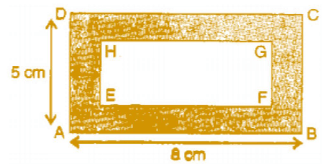And reduced breadth = 5 - (1.5 + 1.5] = 5 - 3 = 2 cm
Area of margin = Area of cardboard (ABCD) - Area of cardboard (EFGH)
= (AB x AD) - (EF x EH)
= (8 x 5) - (5 x 2)
= 40 - 10
= 30 cm2

Thus, total area of margin is 30 cm2.

Question 4:

A verandah of width 2.25 m is constructed all along outside a room which is 5.5 m long and 4 m wide. Find:

(i) the area of the verandah.

(ii) the cost of cementing the floor of the verandah at the rate of Rs 200 per m2

(i) The length of room = 5.5 m
Width of the room = 4 m

The length of room with verandah = 5.5 + 2.25 + 2.25 = 10 m

The width of room with verandah = 4 + 2.25 + 2.25 = 8.5 m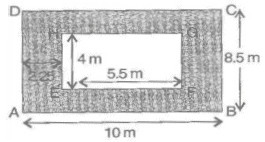Area of verandah = Area of room with verandah – Area of room without verandah

= Area of ABCD – Area of EFGH

= (AB x AD) – (EF x EH)

= (10 x 8.5) – (5.5 x 4)

= 85 – 22

= 63 m

(ii) The cost of cementing 1 m2 the floor of verandah = Rs 200

The cost of cementing 63 m2 the floor of verandah = 200 x 63 = Rs 12,600

Question 5:

A path 1 m wide is built along the border and inside a square garden of side 30 m. Find:

(i) the area of the path.

(ii) the cost of planting grass in the remaining portion of the garden at the rate of Rs 40 per m2

(i) Side of the square garden = 30 m and

Width of the path along the border = 1 m

Side of square garden without path = 30 – (1 + 1) = 30 – 2 = 28 m

Now Area of path = Area of ABCD - Area of EFGH
= (AB x AD) - (EF x EH)
= (30 x 30) - (28 x 28)
= 900 - 784
= 116 m2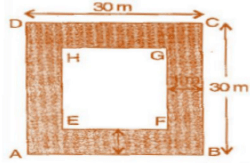(ii) Area of remaining portion = 28 x 28 = 784 m
The cost of planting grass in 1 m2 of the garden = Rs 40
The cost of planting grass in 784 m2 of the garden = Rs 40 x 784 = Rs 31,360

Question 6:

Two cross roads, each of width 10 m, cut at right angles through the centre of a rectangular park of length 700 m and breadth 300 m and parallel to its sides. Find the area of the roads. Also find the area of the park excluding cross roads. Give the answer in hectares.

Here, PQ = 10 m and PS = 300 m, EH = 10 m and EF = 700 m And KL = 10 m and KN = 10 m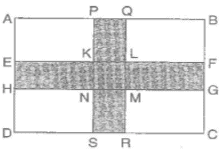Area of roads = Area of PQRS + Area of EFGH - Area of KLMN

[∵ KLMN is taken twice, which is to be subtracted] = (PS x PQ) + (EF x EH)  - (KL x KN)

= (300 x 10) + (700 x 10) - (10 x 10)
= 3000 + 7000 - 100
= 9,900 m2

Area of road in hectares,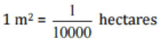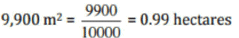Now, Area of park excluding cross roads
= Area of park - Area of road
= (AB x AD) - 9,900 = (700 x 300) - 9,900
= 2,10,000 - 9,900
= 2,00,100 m2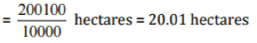Question 7:

Through a rectangular field of length 90 m and breadth 60 m, two roads are constructed which are parallel to the sides and cut each other at right angles through the centre of the fields. If the width of each road is 3 m, find:

(i) the area covered by the roads.

(ii) the cost of constructing the roads at the rate of Rs 110 per m2

(i) Here, PQ = 3 m and PS = 60 m, EH = 3 m and
EF = 90 m and KL = 3 m and KN = 3 m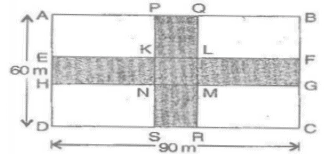Area of roads = Area of PQRS + Area of EFGH - Area of KLMN

[∵  KLMN is taken twice, which is to be subtracted]
= (PS x PQ) + (EF x EH) - (KL x KN)
= (60 x 3)+ (90 x 3) - (3 x 3)
= 180 + 270 - 9
= 441 m2

(ii) The cost of 1 m2 constructing the roads = Rs 110
The cost of 441 m2 constructing the roads = Rs 110 x 441 = Rs 48,510
Therefore, the cost of constructing the roads = Rs 48,510

Question 8:

Pragya wrapped a cord around a circular pipe of radius 4 cm (adjoining figure) and cut off the length required of the cord. Then she wrapped it around a square box of side 4 cm (also shown). Did she have any cord left? (Take π = 3.14)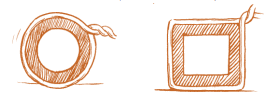Radius of pipe = 4 cm

Wrapping cord around circular pipe = 2πr

= 2 x 3.14 x 4 = 25.12 cm

Again, wrapping cord around a square = 4 x side

= 4 x 4 = 16 cm

Remaining cord = Cord wrapped on pipe – Cord wrapped on square

= 25.12 – 16

= 9.12 cm

Thus, she is left with 9.12 cm cord.

Question 9:

The adjoining figure represents a rectangular lawn with a circular flower bed in the middle. Find: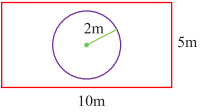(i) the area of the whole land.

(ii) the area of the flower bed.

(iii) the area of the lawn excluding the area of the flower bed.

(iv) the circumference of the flower bed.

Length of rectangular lawn = 10 m, breadth of the rectangular lawn = 5 m
And radius of the circular flower bed = 2 m

(i) Area of the whole land = length x breadth = 10 x 5 = 50 m2

(ii) Area of flower bed = πr2
= 3.14 x 2 x 2 = 12.56 m2

(iii) Area of lawn excluding the area of the flower bed = area of lawn - area of flower bed
= 50 - 12.56
= 37.44 m2

(iv) The circumference of the flower bed = 2πr = 2 x 3.1 4 x 2 = 12.56 m

Question 10:

In the following figures, find the area of the shaded portions: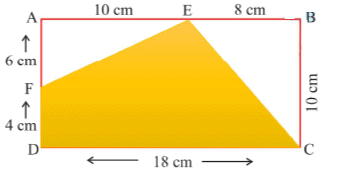(i) Here, AB = 18 cm, BC = 10 cm, AF = 6 cm, AE = 10 cm and BE = 8 cm
Area of shaded portion = Area of rectangle ABCD - (Area of Δ FAE + area of Δ EBC}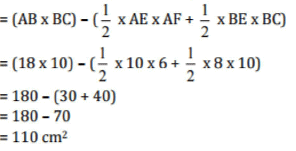(ii) Here, SR = SU + UR = 10 + 10 = 20 cm, QR = 20 cm
PQ = SR = 20 cm
PT = PS - TS =(20 - 10)cm
TS = 10 cm, SU = 10 cm, QR = 20 cm and UR = 10 cm
= Area of square PQRS - Area of ΔQPT - Area of ΔTSU - Area of ΔUQR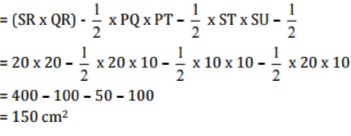Question 11:

Find die area of the equilateral ABCD. Here, AC = 22 cm, BM = 3 cm, DN = 3 cm and BM ⊥ AC, DN ⊥ AC.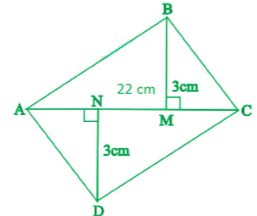Here, AC = 22 cm, BM = 3 cm, DN = 3 cm
Area of quadrilateral ABCDF = Area of Δ ABC + Area of Δ ADC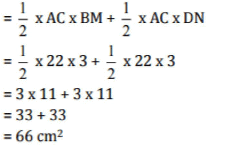Thus, the area of quadrilateral ABCD is 66 cm2.

Offer running on EduRev: Apply code STAYHOME200 to get INR 200 off on our premium plan EduRev Infinity!

,

,

,

,

,

,

,

,

,

,

,

,

,

,

,

,

,

,

,

,

,

;